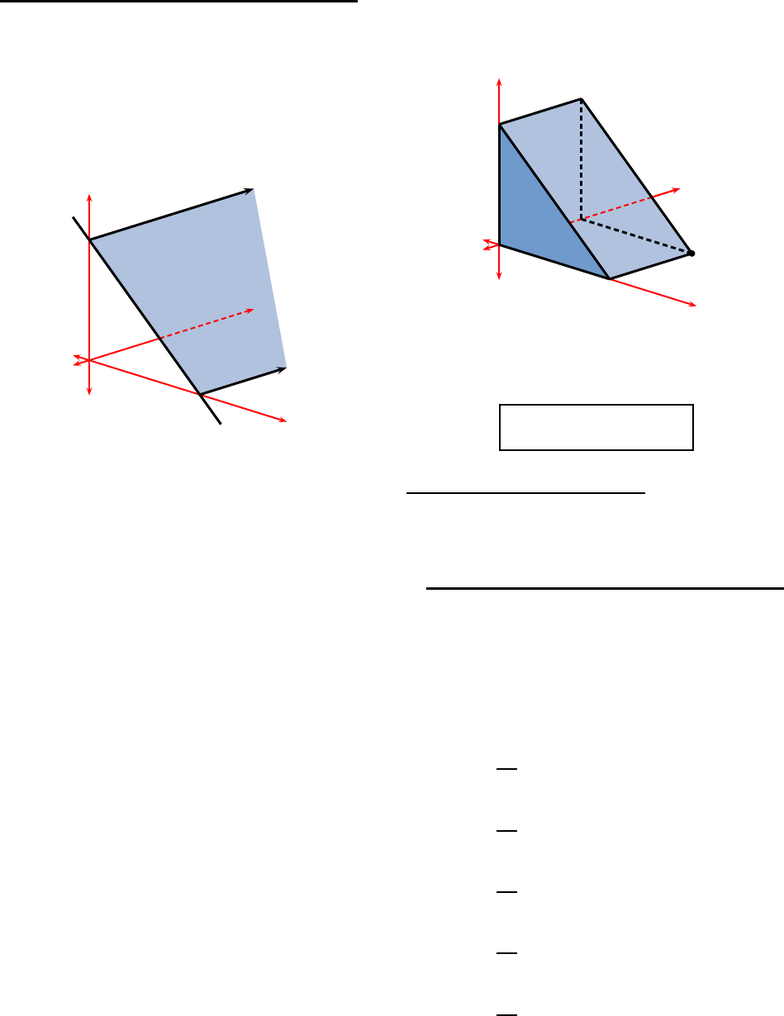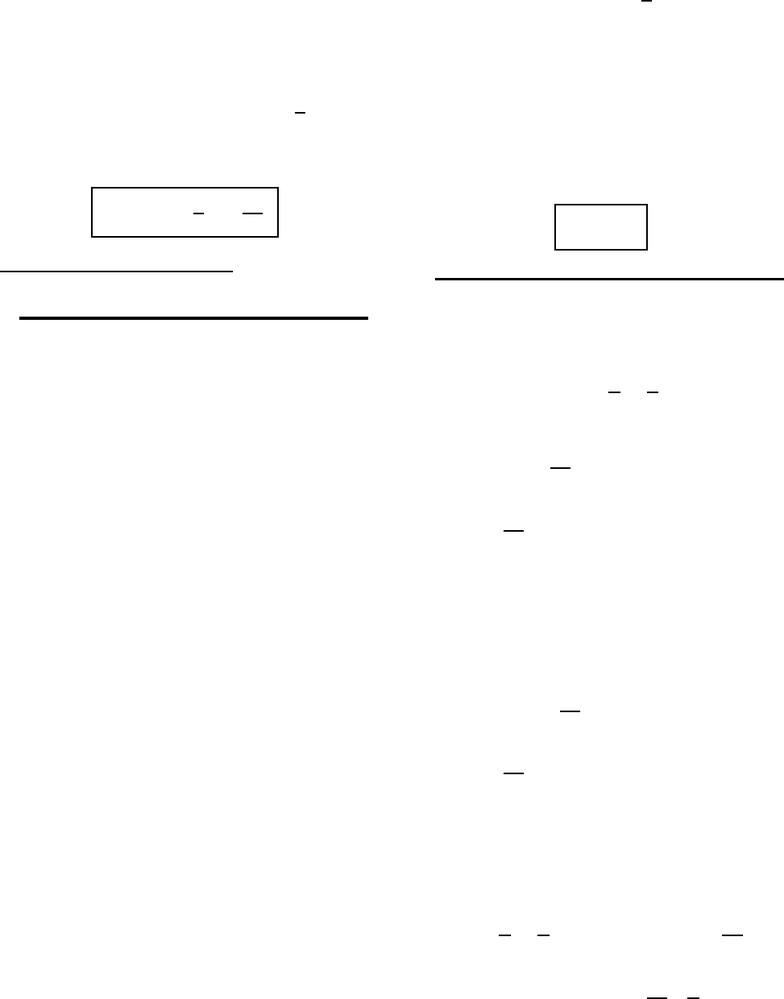# M 408D Chapter Notes - Chapter 15.1: Multiple Integral, Iterated Integral, Iter

26 views5 pages
School
UT-Austin
Department
Mathematics
Course
M 408D
Professoroliver (smo998) – HW 15.1 – rusin – (52440) 1
This print-out should have 9 questions.
Multiple-choice questions may continue on
the next column or page – ﬁnd all choices
001 10.0 points
The graph of the function
z=f(x, y) = 8 x
is the plane shown in
z
8x
y
Determine the value of the double integral
I=Z ZA
f(x, y)dxdy
over the region
A=n(x, y) : 0 x8,0y4o
in the xy-plane by ﬁrst identifying it as the
volume of a solid below the graph of f.
1. I= 126 cu. units
2. I= 128 cu. units correct
3. I= 125 cu. units
4. I= 127 cu. units
5. I= 124 cu. units
Explanation:
The double integral
I=Z ZA
f(x, y)dxdy
is the volume of the solid below the graph of
fhaving the rectangle
A=n(x, y) : 0 x8,0y4o
for its base. Thus the solid is the wedge
z
8
8
x
y
(8,4)
and so its volume is the area of triangular
face multiplied by the thickness of the wedge.
Consequently,
I= 128 cu. units .
keywords: double integral, linear function,
volume under graph, volume, rectangular re-
gion, prism, triangle
002 10.0 points
Determine the value of the iterated integral
I=Z1
0Z2
1
(5 + 2xy)dx dy .
1. I=13
2correct
2. I=21
2
3. I=29
2
4. I=17
2
5. I=25
2
Unlock document

This preview shows pages 1-2 of the document.
Unlock all 5 pages and 3 million more documents.oliver (smo998) – HW 15.1 – rusin – (52440) 2
Explanation:
Integrating with respect to xand holding y
ﬁxed, we see that
Z2
1
(5 + 2xy)dx =h5x+x2yix=2
x=1 .
Thus
I=Z1
0
(5 + 3y)dy =h5y+3
2y2i1
0.
Consequently,
I= 5 + 3
2=13
2.
keywords:
003 10.0 points
Evaluate the double integral
I=Z ZA
(3x+y)dxdy
when
A=n(x, y) : 0 x2,0y1o.
1. I= 9
2. I= 7 correct
3. I= 8
4. I= 10
5. I= 11
Explanation:
Since
A=n(x, y) : 0 x2,0y1o
is a rectangle with sides parallel to the coor-
dinate axes, the value of Ican be found by
interpreting the double integral as the iter-
ated integral
I=Z1
0Z2
0
(3x+y)dxdy .
But now after integration with respect to x
keeping yﬁxed, we see that
Z2
0
(3x+y)dx =h3
2x2+xy i2
0.
Thus
I=Z2
0
(6 + 2y)dy =h6y+y2i1
0.
Consequently,
I= 7 .
004 10.0 points
Evaluate the iterated integral
I=Z4
1Z4
1x
y+y
xdydx .
1. I= 4 ln 15
2
2. I=15
2ln (15)
3. I= 15 ln (4) correct
4. I= 4 ln (15)
5. I= 15 ln 15
2
6. I=15
2ln (4)
Explanation:
Integrating with respect to ykeeping x
ﬁxed, we see that
Z4
1x
y+y
xdy =xln(y) + y2
2x4
1
= (ln(4)) x+15
21
x.
Unlock document

This preview shows pages 1-2 of the document.
Unlock all 5 pages and 3 million more documents.

## Document Summary

Oliver (smo998) hw 15. 1 rusin (52440) Multiple-choice questions may continue on the next column or page nd all choices before answering. The graph of the function z = f (x, y) = 8 x is the plane shown in z y. I = z za f (x, y) dxdy over the region. I = z za f (x, y) dxdy is the volume of the solid below the graph of f having the rectangle. A = n(x, y) : 0 x 8, 0 y 4o for its base. 8 x and so its volume is the area of triangular face multiplied by the thickness of the wedge. I = 128 cu. units keywords: double integral, linear function, volume under graph, volume, rectangular re- gion, prism, triangle. 1 (5 + 2xy) dx dy . correct: i , i , i , i , i = 2 oliver (smo998) hw 15. 1 rusin (52440) Integrating with respect to x and holding y.